## Calculation of the Electron Density of a 2D-Hexagonal Lattice from Diffraction Data (Example).

As an example, the electron density of a 2D-hexagonal lattice is calculated from (X-ray) diffraction data (in relative units). We take the experimental data from the paper Harper et al. 2001, Biophysical Journal, 81, 2693–2706, for fully hydrated aqueous dispersions of the phospholipid DEPE (Di-“elaidoyl”-phosphatidyl-ethanolamine) at 85°C. The first 7 diffraction peaks of the hexagonal phase are given with 1.00 / 0.88 / 0.73 / 0.10 / 0.17 / 0.18 / 0.14, which are the Lorentz- and multiplicity-corrected amplitudes with the signs +/-/-/+/+/+/+. The hexagonal lattice spacing of the lipid at 85°C is 69.9 A.

The parameters for the calculation are entered as input into the Electron density calculator for 2D-hexagonal or 2D-square lattices as shown below: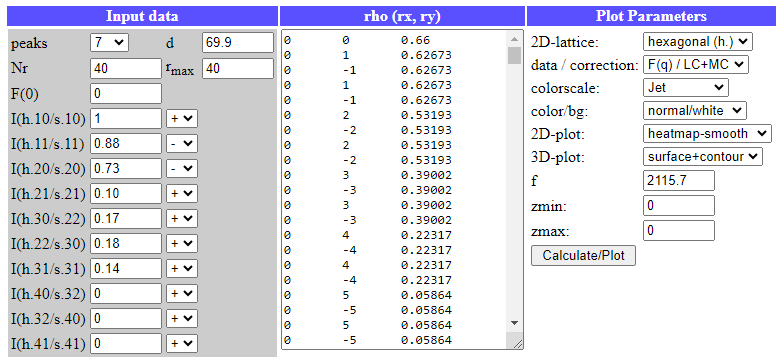The results (e-density of the hexagonal phase) are plotted in 3D and 2D: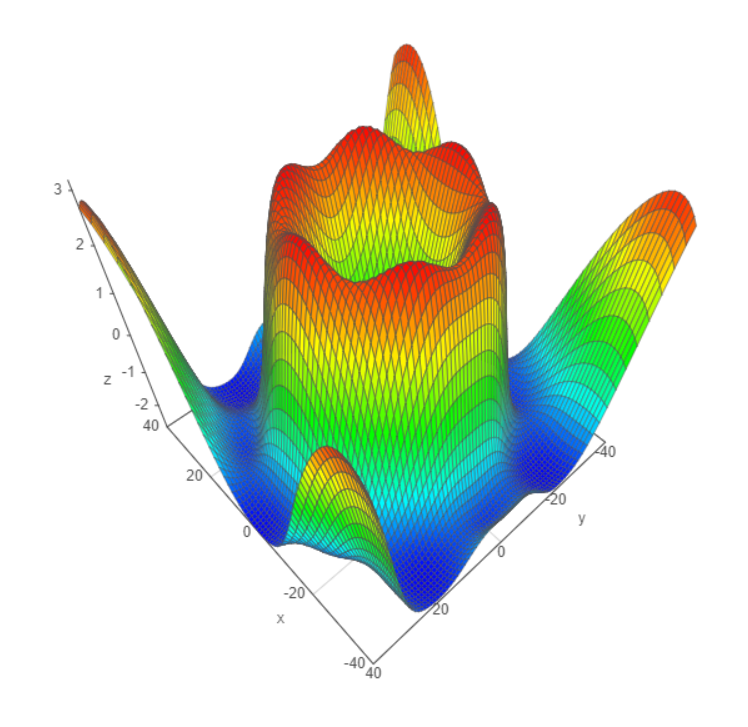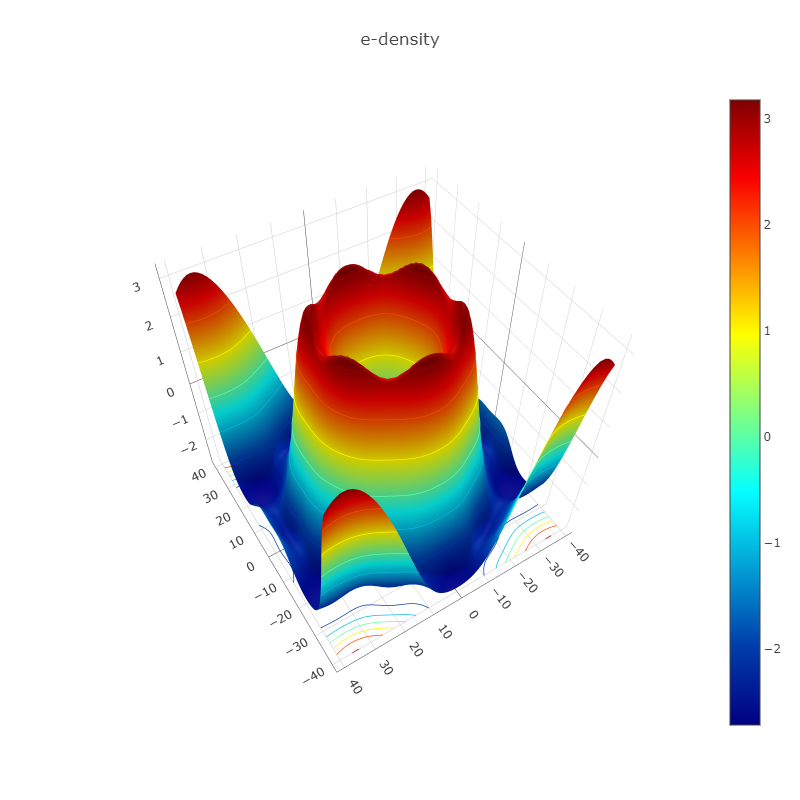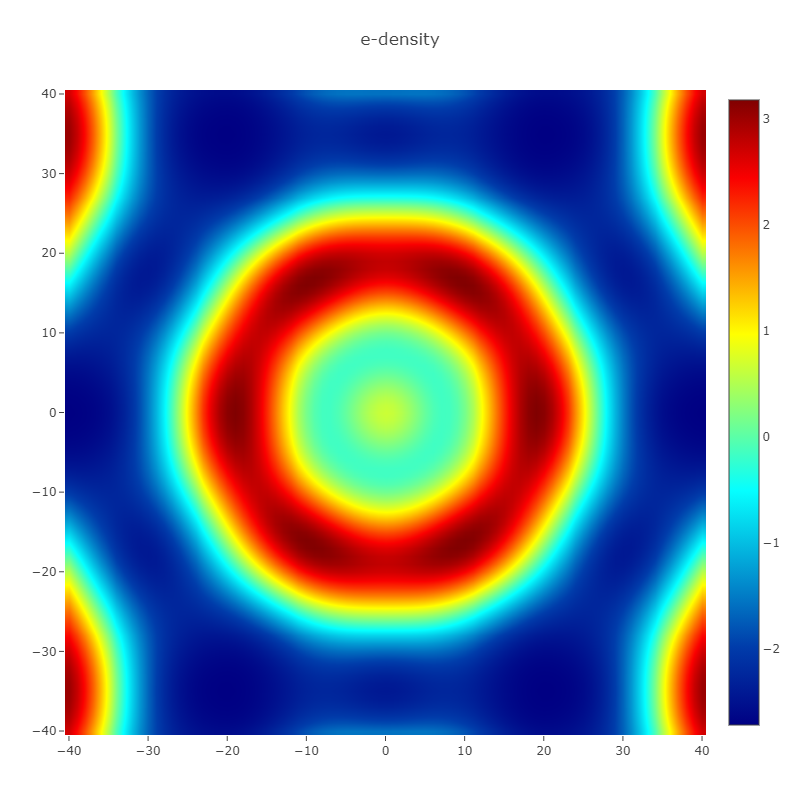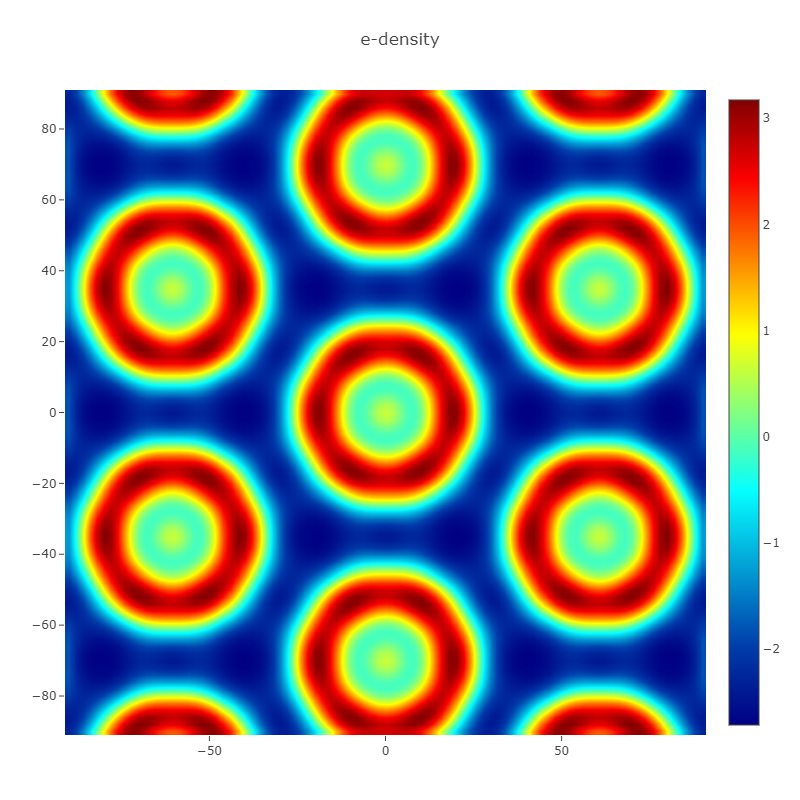Next, we take similar experimental data from the paper Harper et al. 2020, Langmuir, 36, 18, 4908–4916, for fully hydrated aqueous dispersions of the phospholipid POPE (1-palmitoyl-2-oleoyl-sn-glycero-3-phosphatidyl-ethanolamine) with α-tocopherol (mole fraction 0.20) at 40°C. The first 7 diffraction peaks of the hexagonal phase are given with 1.00 / 1.00 / 0.83 / 0.18 / 0.21 / 0.14 / 0.15, which are the Lorentz- and multiplicity-corrected amplitudes with the signs +/-/-/+/+/+/+. The hexagonal lattice spacing of the lipid/tocopherol mixture at 40°C is 74.8 A.

The parameters for the calculation are entered as input into the Electron density calculator for 2D-hexagonal or 2D-square lattices as shown below: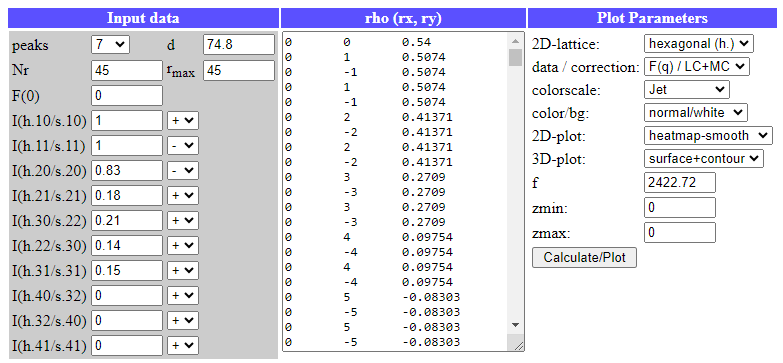The results (e-density of the hexagonal phase) are plotted in 3D and 2D: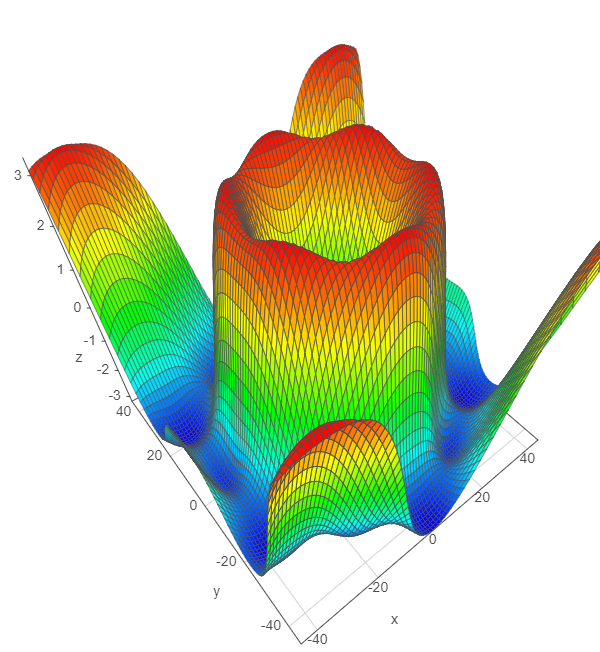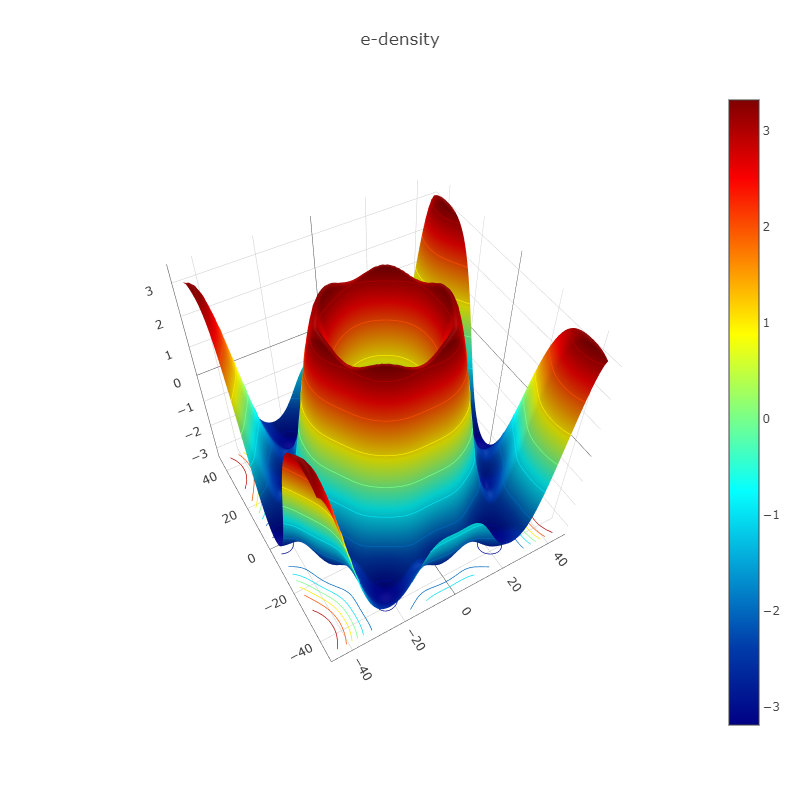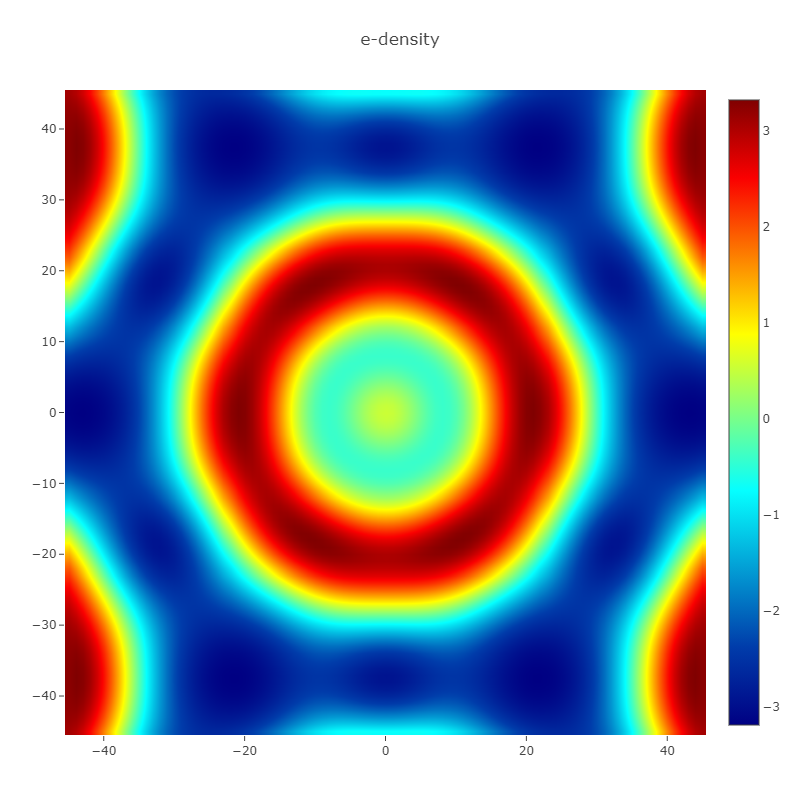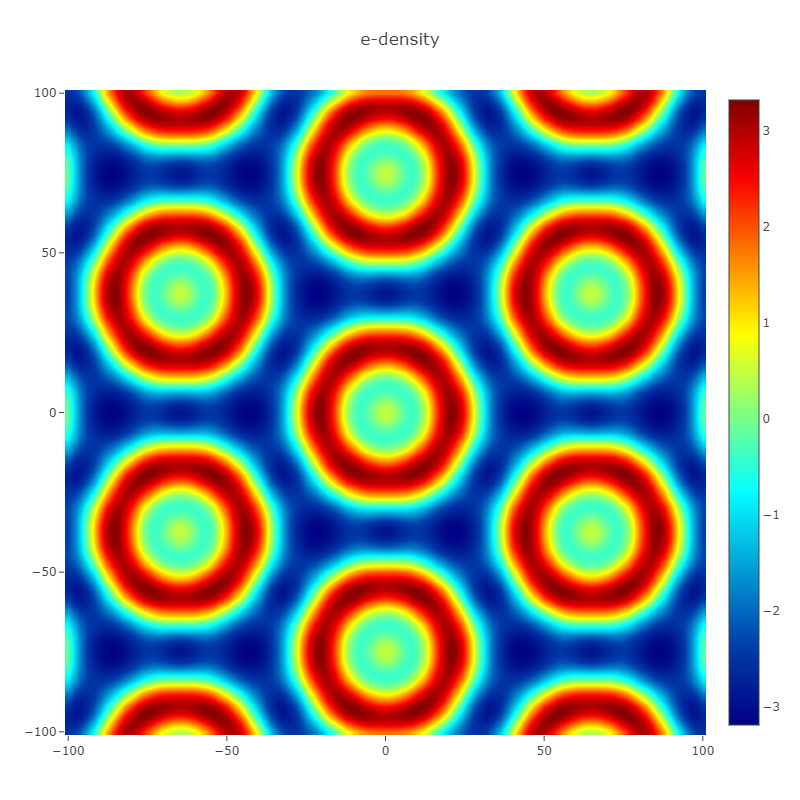Author: M.Kriechbaum, TU-Graz (2020), e-mail: manfred.kriechbaum@tugraz.at